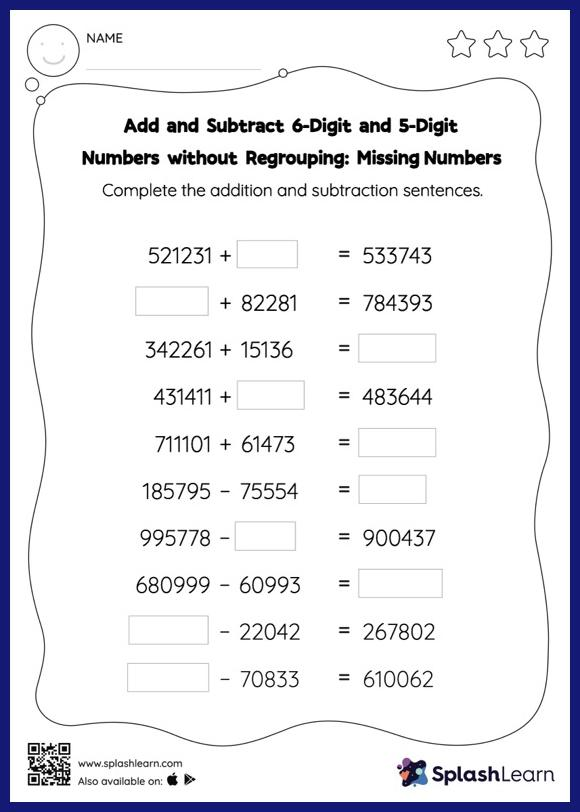# Add and Subtract 6-Digit and 5-Digit Numbers without Regrouping: Missing Numbers Worksheet

Home > Add and Subtract 6-Digit and 5-Digit Numbers without Regrouping: Missing NumbersCan your young mathematician add and subtract 6-digit and 5-digit numbers without regrouping? That's exactly what they do here. To find the missing number on this worksheet, students can utilize the connection between addition and subtraction. In add and subtract 6-digit and 5-digit numbers without regrouping worksheet, they do not need to regroup numbers to reach the solution.